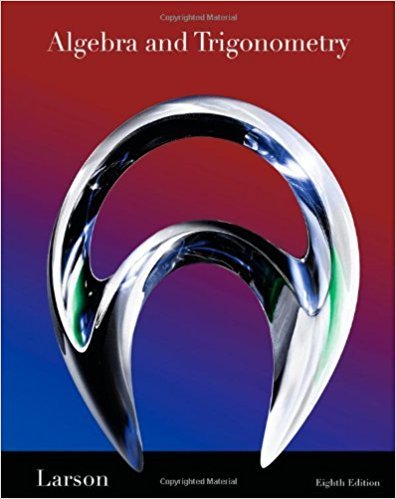×
×

# Solutions for Chapter 9.6: Linear Programming## Full solutions for Algebra and Trigonometry | 8th Edition

ISBN: 9781439048474Solutions for Chapter 9.6: Linear Programming

Solutions for Chapter 9.6
4 5 0 382 Reviews
16
0
##### ISBN: 9781439048474

Chapter 9.6: Linear Programming includes 52 full step-by-step solutions. Since 52 problems in chapter 9.6: Linear Programming have been answered, more than 49205 students have viewed full step-by-step solutions from this chapter. This expansive textbook survival guide covers the following chapters and their solutions. Algebra and Trigonometry was written by and is associated to the ISBN: 9781439048474. This textbook survival guide was created for the textbook: Algebra and Trigonometry, edition: 8.

Key Math Terms and definitions covered in this textbook
• Block matrix.

A matrix can be partitioned into matrix blocks, by cuts between rows and/or between columns. Block multiplication ofAB is allowed if the block shapes permit.

A sequence of steps (end of Chapter 9) to solve positive definite Ax = b by minimizing !x T Ax - x Tb over growing Krylov subspaces.

• Covariance matrix:E.

When random variables Xi have mean = average value = 0, their covariances "'£ ij are the averages of XiX j. With means Xi, the matrix :E = mean of (x - x) (x - x) T is positive (semi)definite; :E is diagonal if the Xi are independent.

• Determinant IAI = det(A).

Defined by det I = 1, sign reversal for row exchange, and linearity in each row. Then IAI = 0 when A is singular. Also IABI = IAIIBI and

• Exponential eAt = I + At + (At)2 12! + ...

has derivative AeAt; eAt u(O) solves u' = Au.

• Fast Fourier Transform (FFT).

A factorization of the Fourier matrix Fn into e = log2 n matrices Si times a permutation. Each Si needs only nl2 multiplications, so Fnx and Fn-1c can be computed with ne/2 multiplications. Revolutionary.

• Free columns of A.

Columns without pivots; these are combinations of earlier columns.

• Iterative method.

A sequence of steps intended to approach the desired solution.

• Krylov subspace Kj(A, b).

The subspace spanned by b, Ab, ... , Aj-Ib. Numerical methods approximate A -I b by x j with residual b - Ax j in this subspace. A good basis for K j requires only multiplication by A at each step.

• Least squares solution X.

The vector x that minimizes the error lie 112 solves AT Ax = ATb. Then e = b - Ax is orthogonal to all columns of A.

• Network.

A directed graph that has constants Cl, ... , Cm associated with the edges.

• Nilpotent matrix N.

Some power of N is the zero matrix, N k = o. The only eigenvalue is A = 0 (repeated n times). Examples: triangular matrices with zero diagonal.

• Nullspace N (A)

= All solutions to Ax = O. Dimension n - r = (# columns) - rank.

• Particular solution x p.

Any solution to Ax = b; often x p has free variables = o.

• Rank r (A)

= number of pivots = dimension of column space = dimension of row space.

• Row picture of Ax = b.

Each equation gives a plane in Rn; the planes intersect at x.

• Spectral Theorem A = QAQT.

Real symmetric A has real A'S and orthonormal q's.

• Vandermonde matrix V.

V c = b gives coefficients of p(x) = Co + ... + Cn_IXn- 1 with P(Xi) = bi. Vij = (Xi)j-I and det V = product of (Xk - Xi) for k > i.

• Vector v in Rn.

Sequence of n real numbers v = (VI, ... , Vn) = point in Rn.

• Volume of box.

The rows (or the columns) of A generate a box with volume I det(A) I.

×